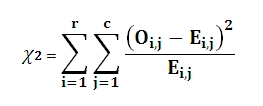# SQL Server CHISQ.TEST function

Updated: 6 August 2010
Use CHITEST_q to calculate the Pearson chi-square test for independence. CHITEST_q returns the value from the chi-square (χ2) distribution for the statistic and the appropriate degrees of freedom.  Calculate the chi-square statistic (χ2) directly using the CHISQ or the CHISQ_q function.

The chi-square statistic is calculated by finding the difference between each observed and theoretical frequency for each possible outcome, squaring them, dividing each by the theoretical frequency, and taking the sum of the results. A second important part of determining the test statistic is to define the degrees of freedom of the test: this is essentially the number of squares errors involving the observed frequencies adjusted for the effect of using some of those observations to define the expected frequencies.

Given the test statistic and the degrees of freedom, the test value is returned by the regularized gamma function Q(a, x) where:

a             is the degrees of freedom divided by 2
x              is χ2 statistic divided by 2

CHITEST_q automatically calculates the expected results and the degrees of freedom

The value of the test statistic isWhere
r              is the number of rows
c              is the number of columns
O             is the Observed result
E              is the Expected result

Syntax
SELECT [wctStatistics].[wct].[CHITEST_q] (
<@Actual_range_RangeQuery, nvarchar(4000),>)
Arguments
@Actual_range_RangeQuery
the select statement, as text, used to determine the actual, or observed, results to be used in the calculation.
Return Types
float
Remarks
·         CHITEST_q is designed for de-normalized tables. For normalized tables, use the CHITESTN_q function.
·         CHITEST_q automatically calculates the expected values and the degrees of freedom.
·         For simpler queries, consider using the CHITEST function.
·         CHITEST_q = CHIDIST(χ2, df), where df = (r-1)(c-1), r>1, c>1
·         To calculate the test statistic, use the CHISQ_q function
·         No GROUP BY is required for this function even though it produces aggregated results.
Examples
In this hypothetical situation, we want to determine if there is an association between population density and the preference for a sport from among baseball, football, and basketball. We will use the CHITEST_q function to perform the chi-squared test.
CREATE TABLE #chi(
[Sport] [varchar] (20)        NOT NULL,
[Rural] [float]               NOT NULL,
[Suburban] [float]            NOT NULL,
[Urban] [float]               NOT NULL
)
INSERT INTO #CHI VALUES ('Baseball',60,43,35)
INSERT INTO #CHI VALUES ('Football',52,48,28)

SELECT wct.CHITEST_q('SELECT Rural,Suburban,Urban FROM #chi')
This produces the following result
----------------------
0.000162912223138266

(1 row(s) affected)

### SupportCopyright 2008-2023 Westclintech LLC         Privacy Policy        Terms of Service